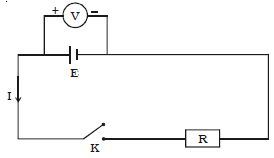Physics

# Determination of Internal Resistance of a Cell Using VoltmeterThe potentiometer is a device used to measure the internal resistance of a cell and is used to compare the e.m.f. of two cells and potential difference across a resistor.

Materials Required: a potentiometer, a battery, (or eliminator) two one way key, a rheostat of low resistance, a galvanometer, a high resistance box, a fractional resistance box, an ammeter a voltmeter, a cell a jockey, a set square, connecting wires, a piece of sandpaper.

The circuit connections are made as shown in Figure. With key K open, the emf of cell E is found by connecting a high resistance voltmeter across it.

Since the high resistance voltmeter draws only a very feeble current for deflection, the circuit may be considered as an open circuit. Hence the voltmeter reading gives the emf of the cell. A small value of resistance R is included in the external circuit and key K is closed. The potential difference across R is equal to the potential difference across the cell (V).Fig: Internal resistance of a cell using voltmeter

The potential drop across R, V = IR … (1)

Due to internal resistance r of the cell, the voltmeter reads a value V, less than the emf of cell.

Then V = E – Ir or Ir = E−V … (2)

Dividing equation (2) by equation (1)

Ir/IR = (E-V)/V or, r = (E-V)/V * R

Since E, V, and R are known, the internal resistance r of the cell can be determined.

Procedure

• The connections should be according to the diagram shown above. Clean the ends of the connecting wires with sandpaper and make a tight connection, tighten the plug of the resistance box. Using sandpaper cleans the ends of the connecting wires and make sure that the connections are tight.
• The e.m.f of the cell and battery is more than that of the cell. Check the emf of the battery and cell and see the emf of the battery is more than that of the given cell otherwise null or balance point won’t be obtained (E’ >E). If it is not then the null point won’t be obtained.
• Take maximum current from battery, making rheostat resistance small. Rheostat resistance can be made minimum by taking maximum current from the battery.
• Insert the plug key k, and adjust the rheostat so that a null point is obtained on the fourth wire of the potentiometer. To check if the circuit connections are correct, the galvanometer deflections should be in the opposite direction.
• Insert the 2000 ohm plug in its position in the resistance box and obtain a null point by slightly adjusting the jockey. Take the small resistance between 1-5 ohm from resistance box R connected in parallel with the cell.
• Slide the jockey and obtain the null point. Measure the balancing length. Take out a small resistance from the resistance box R connected in parallel with cell.
• Slide the jockey along the potentiometer wire and obtain a null point. Record your observation.

Precautions

• The e.m.f of the cell should be lesser than the battery.
• Ensure the current is passed only while obtaining the null point.
• The rheostat should be placed.
• During the experiment, the cell should not be disturbed.
• There shouldn’t be rubbing of jockey against potentiometer wire.